Here are the best pictures I have made. The C code uses fourth order Runge-Kutta with variable Δt. See Weisstein’s note on Runge-Kutta for what you need to know to write the code. (See at least these pictures for clues as to why it works.) Here are comments on the accuracy of the method. 259818 steps were used. This was computed with the Pentium’s 64 significant bits. This Java code (explained here) reads data files produced by the C code and produces .png files which are shown below.

Each picture is for 8 time units. White dots on the curve are where the time has an integral value. Labels for times 35 thru 40 are confusing. At t = 37 and t = 38 the 5 mass (yellow) lands on the track of the 3 mass (turquois). At t = 47 the 5 mass is just back to where it was at about t = 40.6. The greatest numerical distress occurs about 15.7 which event is notable in the picture. The 3 mass is off screen between t = 49 and t = 57 after which it makes its last encounter with the other two which have already begun their final perpetual pas de deux. It passes between the other two in such a phase so as to extract enough of their potential energy to be ejected from the system.

Legend:
 mass color 3 turquois 4 magenta 5 yellow
 0<=t<8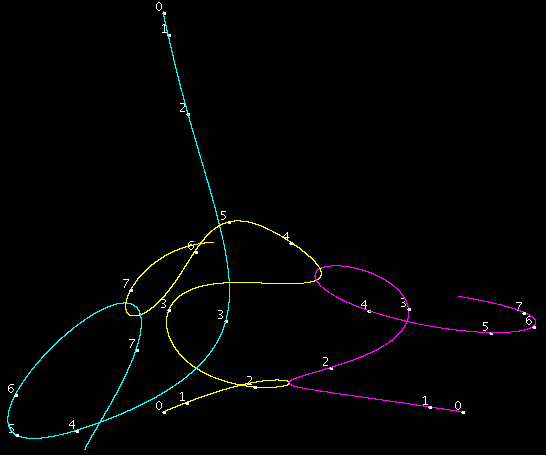at t = 1.8794ke = 2035Δt = 4*10−7 at t = 3.8ke = 324Δt = 6.2*10−6 at t = 6.898ke = 140Δt = 1.3*10−5 8<=t<16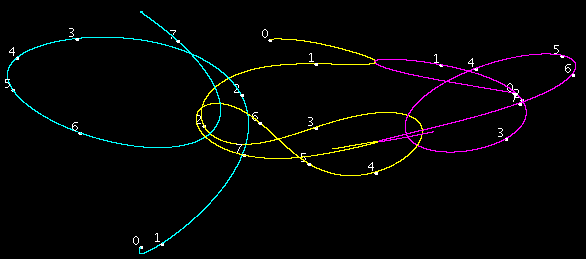at t = 8.76ke = 2341Δt = 3.2*10−7 at t = 15.82992ke = 48249 (Wow!)Δt = 3.4*10−9 16<=t<24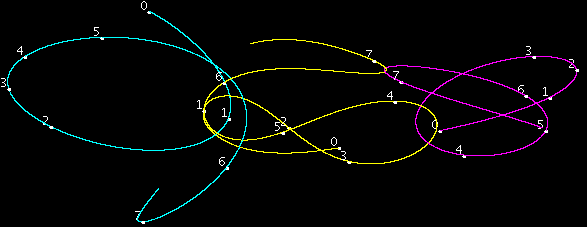at t = 22.965ke = 1137Δt = 9.3*10−7 24<=t<32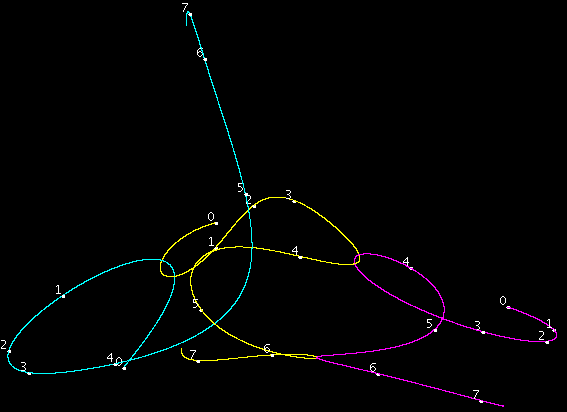at t = 29.8015ke = 7153Δt = 5.9*10−8 32<=t<40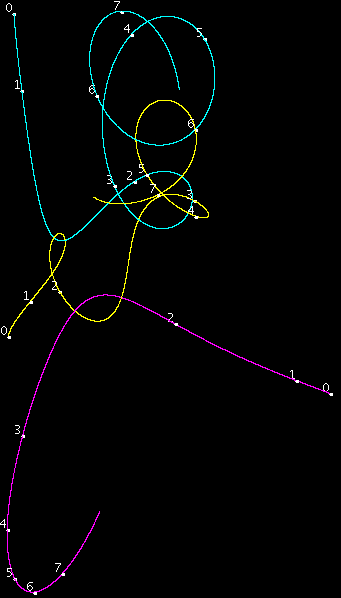at t = 33.6715ke = 213Δt = 7.6*10−6 40<=t<48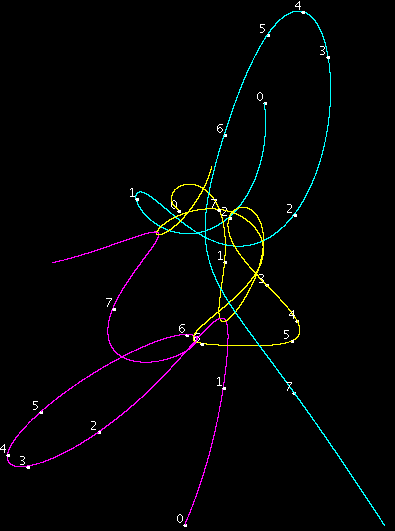at t = 41.209ke = 619Δt = 2.4*10−6 at t = 46.015ke = 596Δt = 2.5*10−6 at t = 47.371ke = 1189Δt = 8.7*10−7 48<=t<56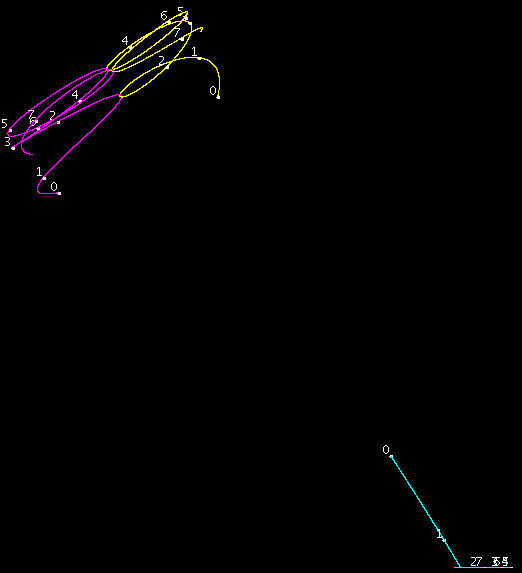at t = 52.116ke = 1513Δt = 6*10−7 etc. 56<=t<62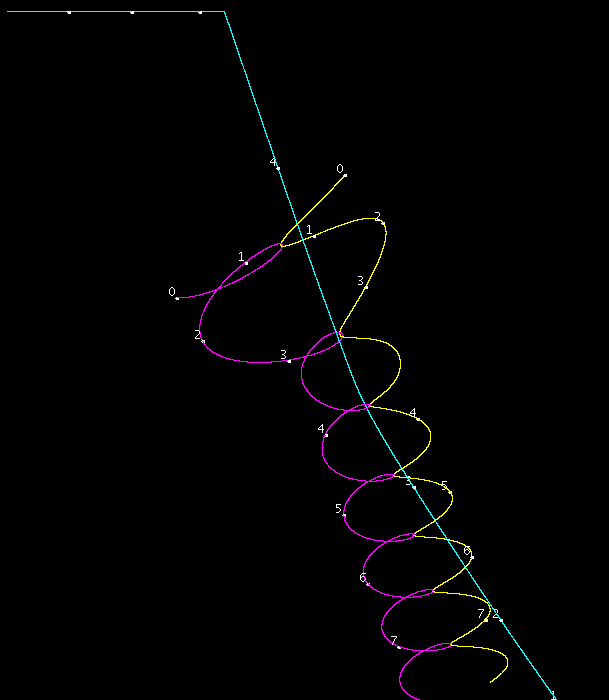at t = 59.777ke = 2662Δt = 6.2*10−8 at t = 60.6335ke = 3067Δt = 6.2*10−8 at t = 61.494ke = 3126Δt = 3.1*10−8
Accuracy